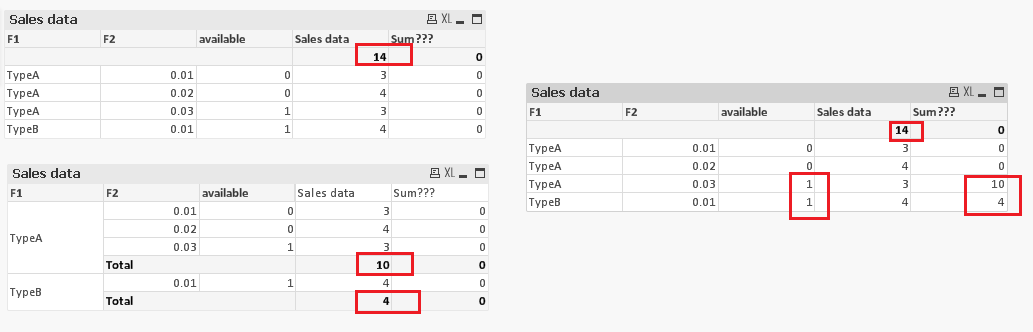# QlikView Scripting

Discussion Board for collaboration on QlikView Scripting.

Announcements

#### Breathe easy -- you now have more time to plan your next steps with Qlik! QlikView 11.2 Extended Support is now valid through December 31, 2020. Click here for more information.Not applicable

## Calculation in straight table

Dear Community,

As shown in the sample document, how can show a new column where its available= 1 and sum of that particular type?

so I would like to see sum of typeA, 3+4+3 = 10, sum of typeB = 4 and so on...

Thanks and best regards,

Chanel

Tags (3)
1 Solution

Accepted Solutions
Not applicable

## Re: Re: Calculation in straight table

Hi chanel,

I am not sure why you need such output where you can easily get with partial sum features.

anyways PFA I have done in all 3 forms may be helpful for you.15 Replies
Not applicable

Here it is

Not applicable

## Re: Calculation in straight table

Try this:

Sum({\$<available={1}>}F2*F3)Not applicable

## Re: Calculation in straight table

You can use the following for calculating sum:

=Sum({\$<available = {1}, F1 = {'Type A'}>} F2*F3)

Hope this helps!

Not applicable

## Re: Calculation in straight table

Please take a look at the attachment. I've few questions. Do you just want to show the TypeA in the chart?

Do you just want to show the row where available =1, what about available =0 do you want that ignored in the row in the st Table?Not applicable

## Re: Calculation in straight table

Thanks for the suggestion, but I want to get sum of TypeA which is 3+4+3 = 10, as well as sum of TypeB = 4 in the new column.Not applicable

## Re: Calculation in straight table

Thanks for the suggestion, is closer to solution now, but I want to get sum of TypeA which is 3+4+3 = 10. Is it possible to make it?Not applicable

## Re: Calculation in straight table

Thanks for helping but sorry, the formula is not working with the " F2*F3) “Not applicable

## Re: Calculation in straight table

Thanks for your questions and suggestion. I want to show the row where available = 1, and sum of the type in that row where available = 1.

so in the new column, i would like to see sum of TypeA which is 3+4+3 = 10 and TypeB = 4.

Thanks and best regards,

ChanelNot applicable

## Re: Calculation in straight table

Hi Chanel,

Does this do what you need it to do...

If(F1='TypeA',sum({<F1={'TypeA'}>}TOTAL F2*F3),sum({<F1={'TypeB'}>}TOTAL F2*F3))

Julian

Community Browser